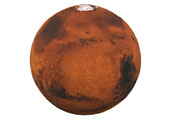OCR GCSE - Astronomy

Page 1

 1. Two satellites compare the gravity of Earth and Mars by orbiting the planets at the same radius of orbit. Measurements from the satellites show that Mars has a smaller gravitational field strength than the Earth.This is because... A) the mass of Mars is smaller. B) the radius of Mars is smaller. C) the radius of Mars is larger. D) the density of Mars is smaller. ? A B C D 2. Which of the following gives the sizes of astronomical objects in order of increasing size? A) Solar system, universe, galaxy. B) Universe, galaxy, solar system. C) Solar system, galaxy, universe. D) Galaxy, solar system, universe. ? A B C D 3. What is the name of our own galaxy containing the Sun? A) The Andromeda galaxy. B) The Milky Way galaxy. C) The Orion galaxy. D) The Hubble galaxy. ? A B C D 4. Which of these statements is correct? A). Planets orbit moons, planets orbit stars. B). Moons orbit planets, stars orbit planets. C). Moons orbit stars, stars orbit planets. D). Moons orbit planets, planets orbit stars. ? A B C D 5. How many planets are there in our solar system? (ignoring dwarf planets). A) 7 B) 8 C) 9 D) 10 ? A B C D 6. Which of these statements best describes the releationship between the orbital speed of a planet and the distance from the Sun? A) The further the distance from the Sun, the weaker gravity is and the slower the orbital speed of the planet. B) The further the distance from the Sun, the weaker gravity is and the faster the orbital speed of the planet. C) The further the distance from the Sun, the stronger gravity is and the slower the orbital speed of the planet. D) The further the distance from the Sun, the stronger gravity is and the faster the orbital speed of the planet. ? A B C D 7. Stars that are stable and producing energy from fusion for millions (or billions) of years are called... A) red giant stars. B) brown dwarf stars. C) main sequence stars. D) variable stars. ? A B C D 8. Which of the statements below best describes the equilibrium between forces within a stable star? A) The inward force caused by magnetic attraction is balanced by the outward force caused by thermal expansion from fusion. B) The inward force caused by gravity is balanced by the outward force caused by nuclear fission. C) The inward force caused by combustion is balanced by the outward force caused by nuclear fission. D) The inward force caused by gravity is balanced by the outward force caused by thermal expansion from fusion. ? A B C D 9. Stars that no longer have any nuclear reactions but are still very hot, luminous and small are called.... A) neutron stars. B) brown dwarf stars. C) main sequence stars. D) white dwarf stars. ? A B C D